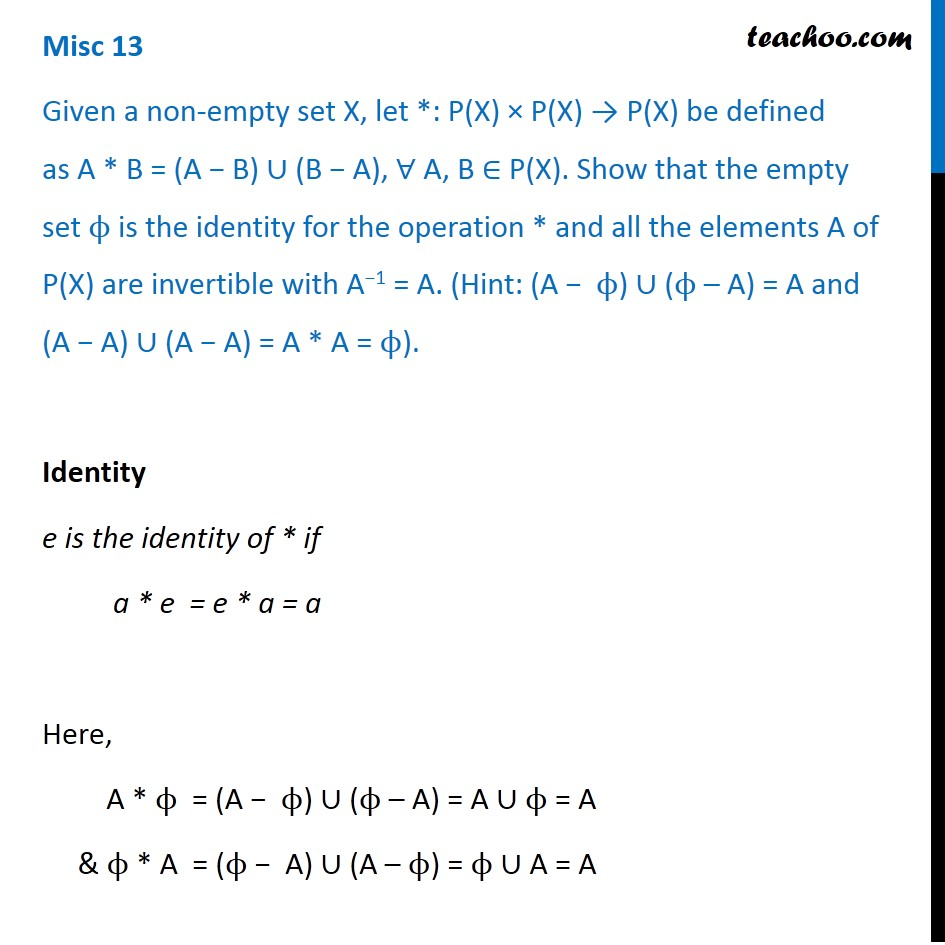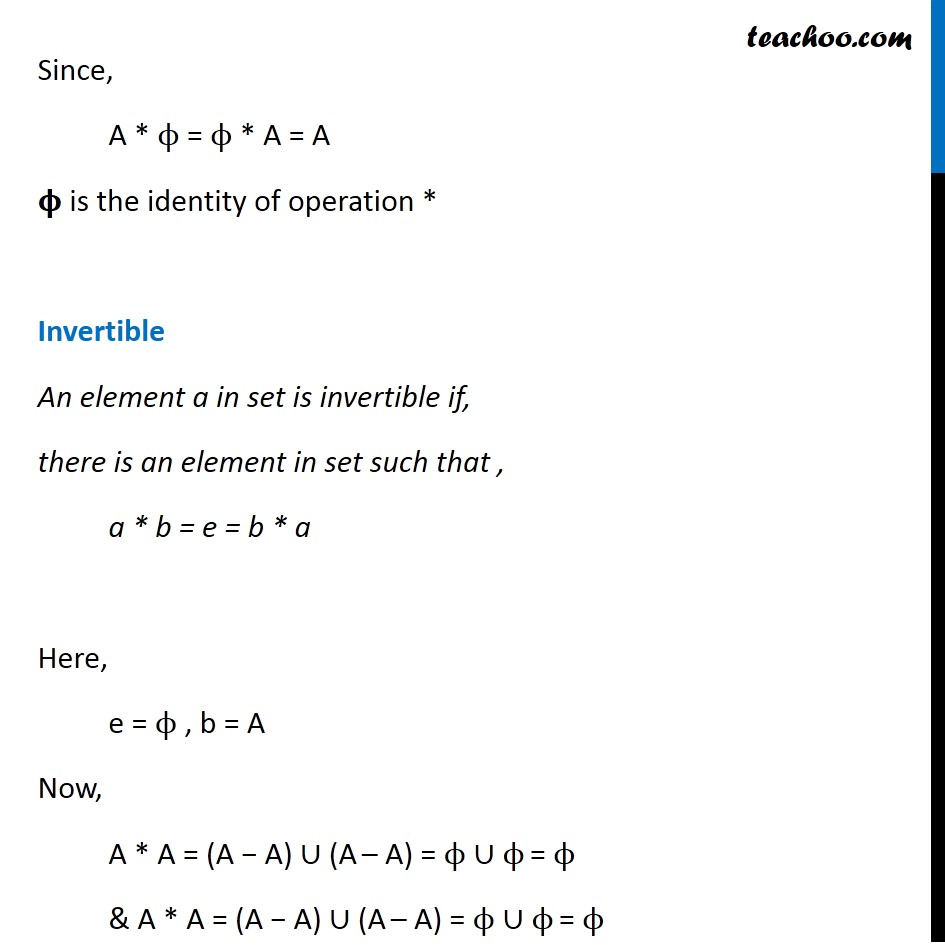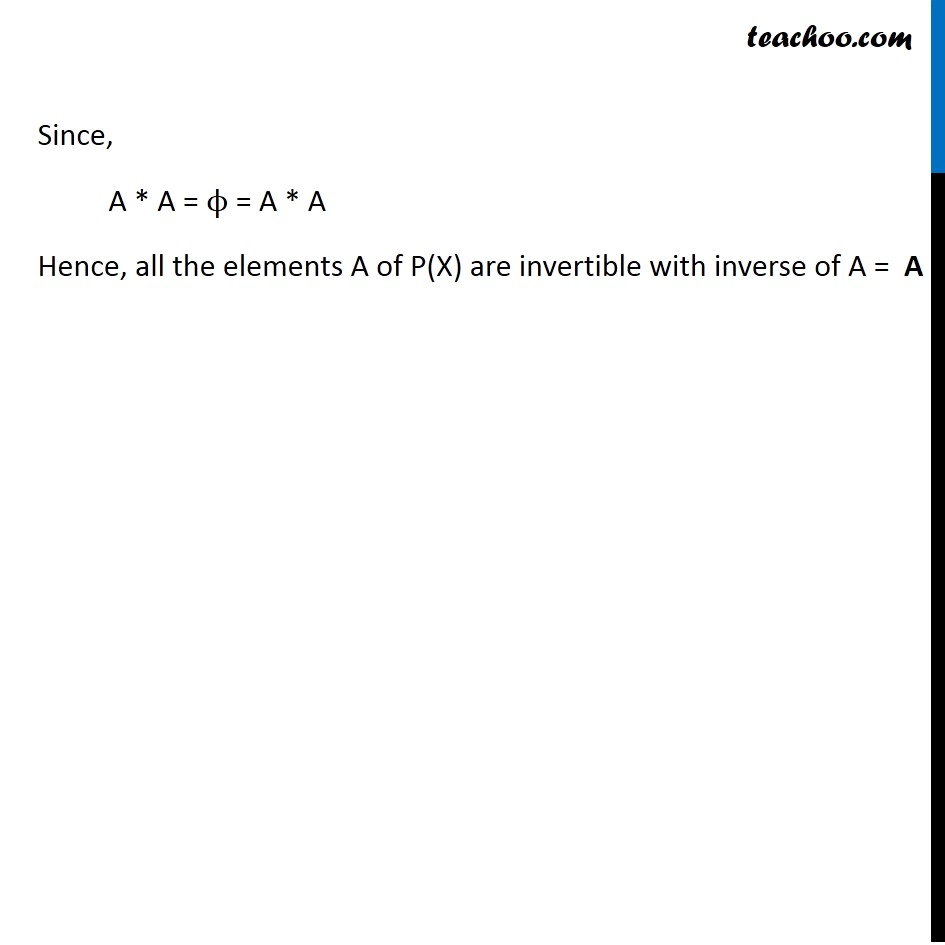Miscellaneous

Chapter 1 Class 12 Relation and Functions
Serial order wiseLearn in your speed, with individual attention - Teachoo Maths 1-on-1 Class

### Transcript

Question 9 Given a non-empty set X, let *: P(X) × P(X) → P(X) be defined as A * B = (A − B) ∪ (B − A), ∀ A, B ∈ P(X). Show that the empty set ϕ is the identity for the operation * and all the elements A of P(X) are invertible with A−1 = A. (Hint: (A − ϕ) ∪ (ϕ – A) = A and (A − A) ∪ (A − A) = A * A = ϕ). Identity e is the identity of * if a * e = e * a = a Here, A * ϕ = (A − ϕ) ∪ (ϕ – A) = A ∪ ϕ = A & ϕ * A = (ϕ − A) ∪ (A – ϕ) = ϕ ∪ A = A Since, A * ϕ = ϕ * A = A 𝛟 is the identity of operation * Invertible An element a in set is invertible if, there is an element in set such that , a * b = e = b * a Here, e = ϕ , b = A Now, A * A = (A − A) ∪ (A – A) = ϕ ∪ ϕ = ϕ & A * A = (A − A) ∪ (A – A) = ϕ ∪ ϕ = ϕ Since, A * A = ϕ = A * A Hence, all the elements A of P(X) are invertible with inverse of A = A